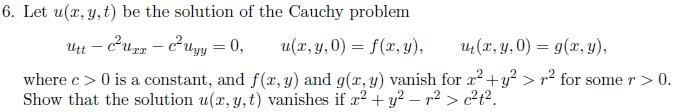# Cauchy Pde Problem!

mmmbohCould someone tell me where to start? I tried separating variables, which got me no where (plus we havent technically learned it), and I tried putting it into a form of D^2U, but I couldnt figure that out either. Please help.

Thank you.

mmmboh
Anyone?

Homework Helper
Have you tried Fourier transforms?

mmmboh
We havent learned that. There must be a more simple way. :S

Homework Helper
What HAVE you done on your course? Once I know this I can select the appropriate technique.

mmmboh
Cauchy problems for the heat and wave equations. Poisson equation, laplace equation, characteristic curves, dirichlet problem, finite difference method, advection in 1d..

Homework Helper
Okay have you done characteristics for 3 variables rather than two?

The way to do this in 1d is to use characteristic co-ordinates.

Mat

mmmboh
No weve only done it for two variables :S...Ill give it a shot, but can you let me know what substitutions to use please? Do i just introduce a third variable equal to like y-ct or what :S

Homework Helper
Hmmm, come to thin about it have you done anything in 2 spacial dimensions? Can you extend what you did on the cauchy problem for the wave eqution?

mmmboh
We looked at the Cauchy problem in 1 spacial dimension. Most of what we have done is in two dimensions total.

Homework Helper
I think the question is saying that it is radially symmetric, do you agree?

mmmboh
Yes thats what it seems like.

Homework Helper
So what is the radially symmetric part of Laplace operator $$\nabla^{2}$$

mmmboh
In polar coordinates, it is (1/r)(d/dr)(rdu/dr)...Am I suppose to take the laplacian of u, and see something?

Homework Helper
Expand that out and denote v=ru, and what equation do you have now?

mmmboh
But u(x,y,t) is not in terms of r, can I write u(r,t) instead and use the laplacian on that? If thats what you mean..or do you mean something else?

I can expand it, and get (1/r)(du/dr)+(d/dr)(du/dr), but there is no u*r in this.

If I let u=v/r, I get v/r^3.

Last edited:
Homework Helper
You should end up with the 1D equation for v, solve this in the usual manner and the problem is easily solved.

mmmboh
I get v/r^3 = 0 :S...I edited my post if you didnt see, can you check if thats what Im suppose to get? (but I still dont yet see how this will show that u vanishes for the given condition, or why we can assume u satisfies laplaces equation).

Thanks for the help btw.

Last edited:
Homework Helper
Just to confirm the equation you should be solving is:

$$\frac{\partial^{2}u}{\partial t^{2}}-c^{2}\left(\frac{\partial^{2}u}{\partial r^{2}}+\frac{2}{r}\frac{\partial u}{\partial r}\right) =0$$

Use $$v(t,r)=ru(r,t)$$ to obtain a 1D wave equation, turn that intop characteristic co-ordinates in the usual way and that will give you your solution.

I am off to bed now.

mmmboh
So do you mean I should I write u=v/r, and insert that into the equation you wrote? Good night, thanks.

If so, I get vtt=0, hope that is right!

Homework Helper
Yes, have you not been doing that?

mmmboh
I wasnt before :S, but now I get I get vtt=0, so I guess I can just integrate that and substitute u in.

Homework Helper
as u=u(t,r) so is v=v(t,r)

mmmboh
I get u(r,t)=a(r)*r*t+r*b(r), where a,b are some functions of r. I dont see how this means u vanished for the given condition though :S

Edit: Oops I didn`t differentiate the v.

Last edited:
Homework Helper
Calculate:

$$\frac{\partial^{2}v}{\partial r^{2}}$$

to get the folowing equation:

$$\frac{\partial^{2}v}{\partial t^{2}}-c^{2}\frac{\partial^{2}v}{\partial r^{2}}=0$$

Then introduce the co-ordinates:

$$\begin{array}{ccc} \alpha & = & r+ct \\ \beta & = & r-ct \end{array}$$

use the condition you have and this should give you the result you need.